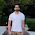## Monday, 15 May 2017

### Facebook's Prophet Model for forecasting

Forecasting is central to data science activities.Facebook's open source forecasting tool 'PROPHET' is available in R and Python. This has been very useful in Web-sites' page view forecasting, road traffic forecasting and in the areas where there is multiple level of seasonality. Prophet is useful in below scenarios-

• Seasonal data ( Hourly, daily,weekly, monthly).
• Data having outliers.
• Data with holidays' information.
• Having multiple trend change points.

Prophet works on additive regressive model with four component.

1. A piece-wise linear or logistic growth curve trend. Prophet automatically detects changes in trends by selecting change points from the data.
2. A yearly seasonal component modelled using Fourier series.
3. A weekly seasonal component using dummy variables.
4. A user-provided list of important holidays.
Here is the example of using prophet model in R.
we take model_data dataset. It must have ds column which is to have dates and other column 'y' which is the values to forecast ( Uni-variate time series). This is how data look like -

running the model -
mod <- prophet::prophet(model_data, weekly.seasonality =F)
There is no weekly seasonality so one of the argument is false.

Preparing op data-set to store result -
future <- make_future_dataframe(mod, periods = 4, freq = 'month')
we want to predict for next 4 data points and on monthly basis. This can be day, week etc.

predicting future values -
forecast <- predict(mod, future)

Visualising result-
forecast <- predict(mod, future)

Here are the forecasted values for the coming months- Yhat are output values with the range of yhat_lower and yhat_upper.

Different component of forecasts can also be plotted like this-

Impact of confidence interval in forecasting-

m1 <- prophet::prophet(n_train, weekly.seasonality =F, interval.width = 0.99)
m2 <- prophet::prophet(n_train, weekly.seasonality =F, interval.width = 0.80)

I have run above 2 models to see impact of having different confidence interval in forecasting-
Here is the result of forecasting using above two-

mean values are same but the variation increases with increase in confidence interval.

This is how, we can use prophet model for time series forecasting!

Here is another time series technique. Which is more related to pure linear regression  and good to know-
Time-Series and Regression Relation

MBA is no longer Masters in business analytics. it's something else. ↙😋 If you want to know-

1.This comment has been removed by the author.

2.how yhat_upper and lower are related to the confidence interval.I read somewhere that yhat_upper and lower (uncertainty levels) determine the confidence interval / the width of the band around predictions. Uncertainty levels are set using interval_width hyperparameter whose default value 80%. This still does not solve my problem.
How can i interpret yhat_upper and lower in terms of % confidence interval?
Thanks

1.I have updated the blog with answer to your question. The value passed in interval.width would decide confidence interval, as you rightly suggested. I hope this answers your question.